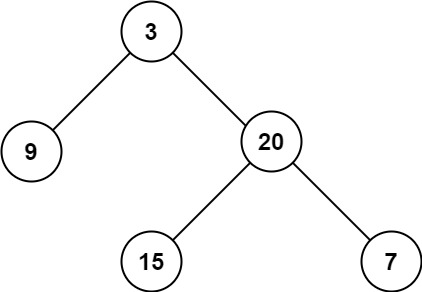## 104. Maximum Depth of Binary Tree

Given the `root` of a binary tree, return its maximum depth.

A binary tree's maximum depth is the number of nodes along the longest path from the root node down to the farthest leaf node.

Example 1:```Input: root = [3,9,20,null,null,15,7]
Output: 3
```

Example 2:

```Input: root = [1,null,2]
Output: 2
```

Example 3:

```Input: root = []
Output: 0
```

Example 4:

```Input: root = 
Output: 1
```

Constraints:

• The number of nodes in the tree is in the range `[0, 104]`.
• `-100 <= Node.val <= 100`

## Rust Solution

``````struct Solution;
use rustgym_util::*;

trait MaxDepth {
fn max_depth(&self) -> i32;
}

impl MaxDepth for TreeLink {
fn max_depth(&self) -> i32 {
if let Some(node) = self {
let node = node.borrow();
return 1 + i32::max(node.left.max_depth(), node.right.max_depth());
}
0
}
}

impl Solution {
fn max_depth(root: TreeLink) -> i32 {
root.max_depth()
}
}

#[test]
fn test() {
let p = tree!(3, tree!(9), tree!(20, tree!(15), tree!(7)));
assert_eq!(Solution::max_depth(p), 3);
}
``````

Having problems with this solution? Click here to submit an issue on github.